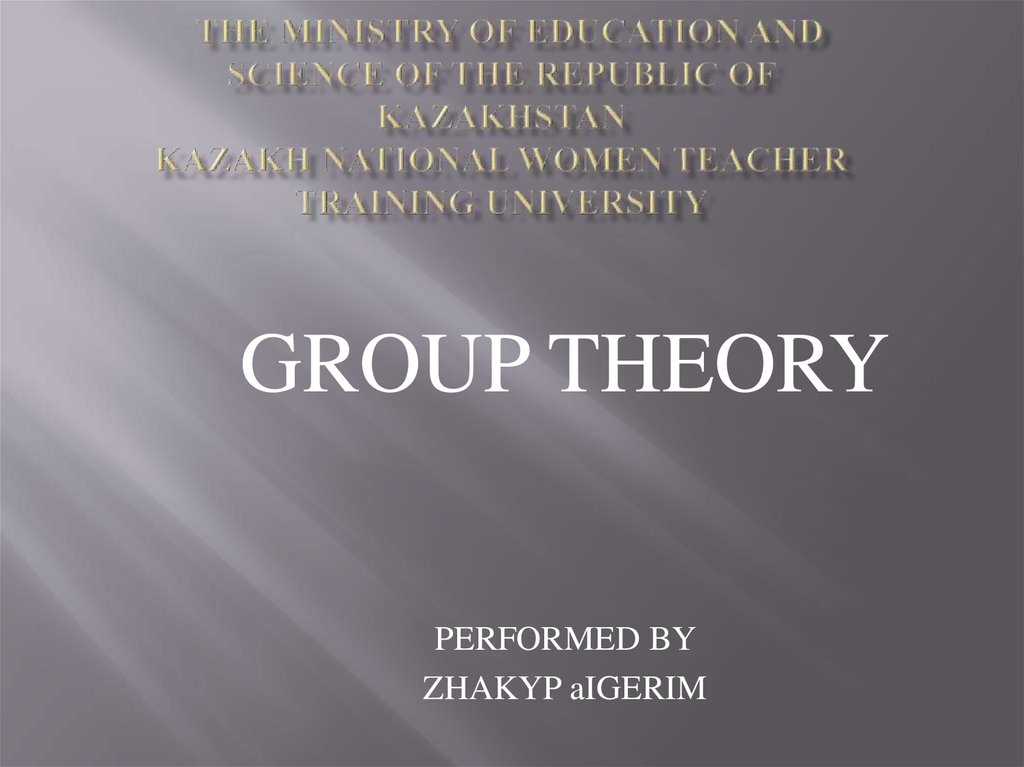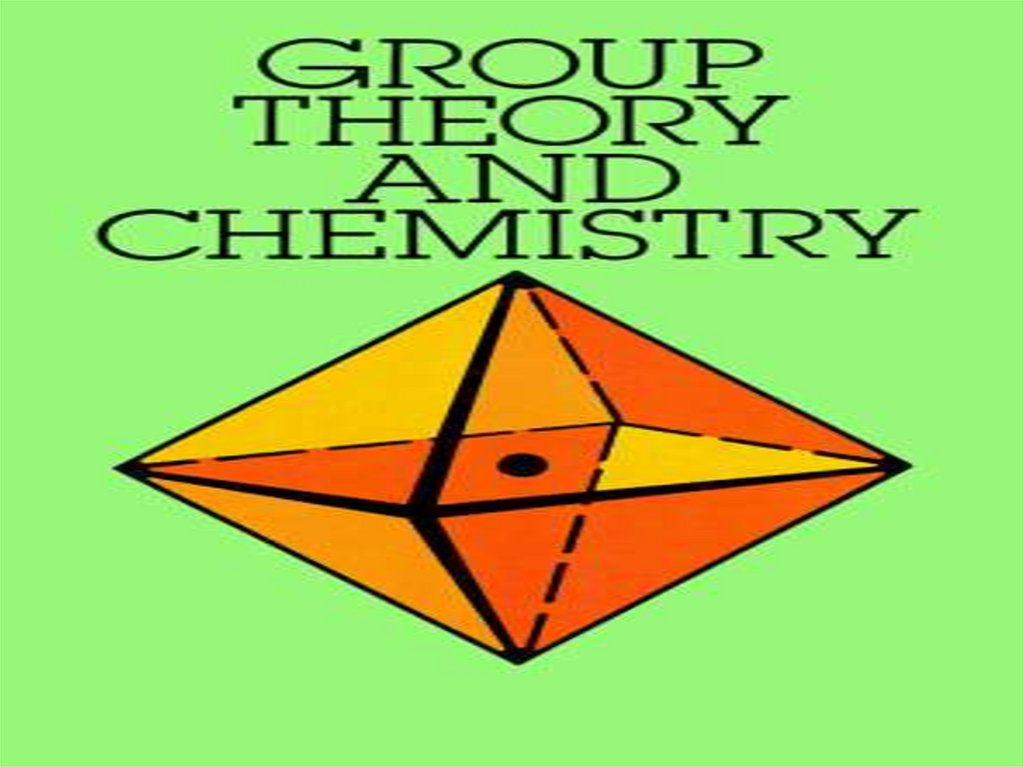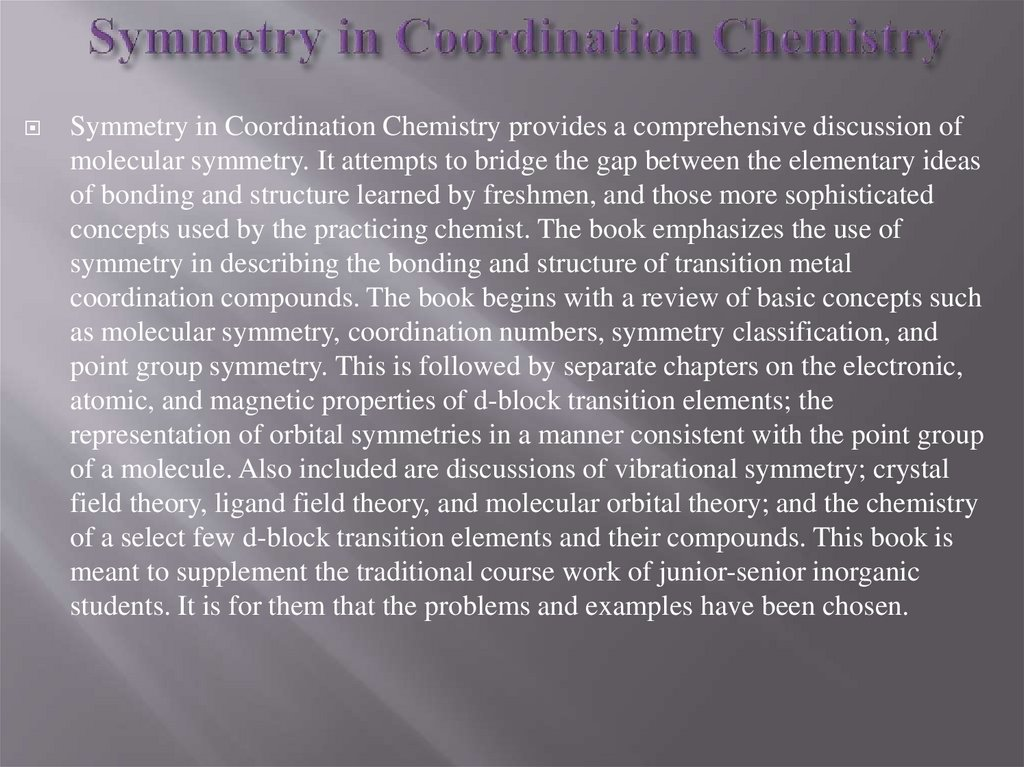# Group theory. Symmetry in coordination chemistry

GROUP THEORY
PERFORMED BY
ZHAKYP aIGERIM

## 3. Symmetry in Coordination Chemistry

provides a comprehensive discussion of
molecular symmetry. It attempts to bridge the gap between the elementary ideas
of bonding and structure learned by freshmen, and those more sophisticated
concepts used by the practicing chemist. The book emphasizes the use of
symmetry in describing the bonding and structure of transition metal
coordination compounds. The book begins with a review of basic concepts such
as molecular symmetry, coordination numbers, symmetry classification, and
point group symmetry. This is followed by separate chapters on the electronic,
atomic, and magnetic properties of d-block transition elements; the
representation of orbital symmetries in a manner consistent with the point group
of a molecule. Also included are discussions of vibrational symmetry; crystal
field theory, ligand field theory, and molecular orbital theory; and the chemistry
of a select few d-block transition elements and their compounds. This book is
meant to supplement the traditional course work of junior-senior inorganic
students. It is for them that the problems and examples have been chosen.

## 4.

Symmetry is important to chemistry because it
undergirds essentially all specific interactions
between molecules in nature (i.e., via the interaction
of natural and human-made chiral molecules with
inherently chiral biological systems). The control of
the symmetry of molecules produced in
modern chemical synthesis contributes to the ability
of scientists to offer therapeutic interventions with
minimal side effects. A rigorous understanding of
symmetry explains fundamental observations
in quantum chemistry, and in the applied areas
of spectroscopy and crystallography. The theory and
application of symmetry to these areas of physical
science draws heavily on the mathematical area
of group theory

## 5.

Molecular symmetry
Molecular symmetry in chemistry describes
the symmetry present in molecules and the classification of
molecules according to their symmetry. Molecular
symmetry is a fundamental concept in chemistry, as it can
be used to predict or explain many of a
molecule's chemical properties, such as its dipole
moment and its allowed spectroscopic transitions. Many
university level textbooks on physical chemistry, quantum
chemistry, and inorganic chemistry devote a chapter to
symmetry.
The predominant framework for the study of molecular
symmetry is group theory. Symmetry is useful in the study
of molecular orbitals, with applications such as the Hückel
method, ligand field theory, and the Woodward-Hoffmann
rules. Another framework on a larger scale is the use
of crystal systems to describe crystallographic symmetry in
bulk materials.
Many techniques for the practical assessment of molecular
symmetry exist, including X-ray crystallography and
various forms of spectroscopy. Spectroscopic notation is
based on symmetry considerations.

## 6.

This group is called
the point group of that
molecule, because the set of
symmetry operations leave
at least one point fixed
(though for some
symmetries an entire axis or
an entire plane remains
fixed). In other words, a
point group is a group that
summarizes all symmetry
operations that all
molecules in that category
have. The symmetry of a
crystal, by contrast, is
described by a space
group of symmetry
operations, which
includes translations in
space.

Point
group
Symmetr
y
operation
s
Simple
description
of typical
geometry
C1
E
no
symmetry,
chiral
Cs
E σh
mirror plane,
no other
symmetry
Ci
Ei
inversion
center
Example 1
Example 2
Example 3

## 9.

(mathematics).
For group theory in social scienspaces, can all be seen as groups
endowed with additionalces, see social group.
In mathematics and abstract algebra, group theory studies
the algebraic structures known as groups. The concept of a
group is central to abstract algebra: other well-known
algebraic
structures,
such
as
rings,
fields,
and vector operations and axioms. Groups recur throughout
mathematics, and the methods of group theory have
influenced many parts of algebra. Linear algebraic
groups and Lie groups are two branches of group theory that
have experienced advances and have become subject areas in
their own right.
Various physical systems, such as crystals and the hydrogen
atom, may be modelled by symmetry groups. Thus group
theory and the closely related representation theory have
many important applications in physics, chemistry,
and materials science. Group theory is also central to public
key cryptography.

## 10.

One of the most important mathematical
achievements of the 20th century was the
collaborative effort, taking up more than 10,000
journal pages and mostly published between
1960 and 1980, that culminated in a
complete classification of finite simple groups.

## 11.

Main classes of groups
Main article: Group (mathematics)
The range of groups being considered has gradually expanded
from finite permutation groups and special examples of matrix groups to
abstract groups that may be specified through
a presentation by generators and relations.